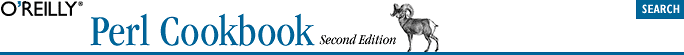home | O'Reilly's CD bookshelfs | FreeBSD | Linux | Cisco | Cisco Exam## 11.5. Taking References to Scalars

### 11.5.3. Discussion

```sub new_anon_scalar {
my \$temp;
return \\$temp;
}```

Dereference a scalar reference by prefacing it with \$ to get at its contents:

```\$sref = new_anon_scalar( );
\$\$sref = 3;
print "Three = \$\$sref\n";
@array_of_srefs = ( new_anon_scalar( ), new_anon_scalar( ) );
\${ \$array } = 6.02e23;
print "\@array contains: ", join(", ", map { \$\$_ } @array ), "\n";```

Notice we put braces around \$array and \$array. If we tried to say \$\$array, the tight binding of dereferencing would turn it into \$array->. It would treat \$array as an array reference and return the element at index zero.

Here are other examples where it is safe to omit the braces:

```\$var        = `uptime`;     # \$var holds text
\$vref       = \\$var;        # \$vref "points to" \$var
if (\$\$vref =~ /load/) {  }    # look at \$var, indirectly
chomp \$\$vref;               # alter \$var, indirectly```

As mentioned in the Introduction, you may use the ref built-in to inspect a reference for its referent's type. Calling ref on a scalar reference returns the string "SCALAR":

```# check whether \$someref contains a simple scalar reference
if (ref(\$someref) ne "SCALAR") {
die "Expected a scalar reference, not \$someref\n";
}```